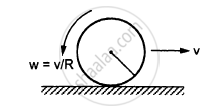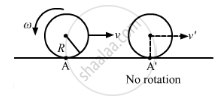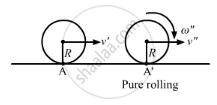Department of Pre-University Education, KarnatakaPUC Karnataka Science Class 11
Advertisement Remove all ads

# A Solid Sphere is Set into Motion on a Rough Horizontal Surface with a Linear Speed ν in the Forward Direction and an Angular Speed ν / R in the Anticlockwise Directions - Physics

Sum

A solid sphere is set into motion on a rough horizontal surface with a linear speed ν in the forward direction and an angular speed ν/R in the anticlockwise directions as shown in the following figure. Find the linear speed of the sphere (a) when it stops rotating and (b) when slipping finally ceases and pure rolling starts.Advertisement Remove all ads

#### Solution

(a)Initial angular momentum about point A,

$L = m\left( v \times R \right) - I\omega$

$= mvR - \frac{2}{5}m R^2 \left( \frac{v}{R} \right)$

$= \frac{3}{5}mvR$

Angular momentum about point A' after it stops rotating,

$L' = mv'R$

As no external torque is applied, angular momentum will be conserved.

Therefore, we have

L = L'

$\Rightarrow mvR = \frac{5}{3}mv'R$

$\Rightarrow v' = \frac{3v}{5}$

(b)Angular momentum about point A after it stops rotating,

$L' = mv'R$

Angular momentum about point A' after it starts pure rolling.

$L'' = I\omega'' + m\left( v'' \times R \right)$

$= \frac{2}{5}m R^2 \left( \frac{v''}{R} \right) + mv'' R$

$= \frac{7}{5}mVR$

As no external torque is applied, angular momentum will be conserved.

Therefore, we have

$L' = L''$

$\Rightarrow m\frac{3V}{5}R = \frac{7}{5}mv'' R$

$\Rightarrow v'' = \frac{3v}{7}$

Is there an error in this question or solution?
Advertisement Remove all ads

#### APPEARS IN

HC Verma Class 11, 12 Concepts of Physics 1
Chapter 10 Rotational Mechanics
Q 85 | Page 200
Advertisement Remove all ads
Advertisement Remove all ads
Share
Notifications

View all notifications

Forgot password?